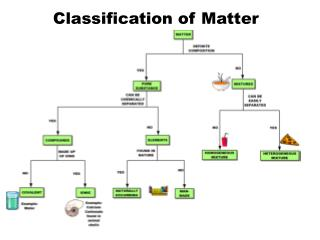DownloadDownload PresentationClassification of Matter

# Classification of Matter

Download Presentation## Classification of Matter

- - - - - - - - - - - - - - - - - - - - - - - - - - - E N D - - - - - - - - - - - - - - - - - - - - - - - - - - -
##### Presentation Transcript

1. Classification of Matter

2. Atomic Theory of Matter • The theory that atoms are the fundamental building blocks of matter reemerged in the early 19th century, championed by John Dalton.

3. Dalton’s Postulates • Each element is composed of extremely small particles called atoms. • All atoms of a given element are identical to one another in mass and other properties, but the atoms of one element are different from the atoms of all other elements. • Compounds result from the chemical combination of a specific ratio of atoms of different elements. • Atoms of an element are not changed into atoms of a different element by chemical reactions; atoms are neither created nor destroyed in chemical reactions.

4. Law of Constant CompositionJoseph Proust (1754–1826)‏ • Also known as the law of definite proportions. • The elemental composition of a pure substance never varies.

5. Law of Multiple Proportions • Two or more different compounds can be made with the same proportion. • Same elements can form different compounds each with a constant composition.

6. Multiple Proportions

7. Law of Conservation of Mass The total mass of substances present at the end of a chemical process is the same as the mass of substances present before the process took place.

8. Subatomic Particles • Protons and electrons are the only particles that have a charge. • Protons and neutrons have essentially the same mass. • The mass of an electron is so small we ignore it.

9. Symbols of Elements Elements are symbolized by one or two letters.

10. Atomic Number The atomic number (Z) - All atoms of the same element have the same number of protons. The mass number (A) – The total number of protons and neutrons in an atom.‏

11. 11 6 12 6 13 6 14 6 C C C C Isotopes • Atoms of the same element with different masses. • Isotopes have different numbers of neutrons.

12. Hydrogen Isotope Activity

13. Average Mass • Because in the real world we use large amounts of atoms and molecules, we use average masses in calculations. • Average mass is calculated from the isotopes of an element weighted by their relative abundances.

14. Practice Problems • Chlorine has two naturally occurring isotopes – Cl35 (isotopic mass of 34.9689 amu) and Cl37 (isotopic mass of 36.9659 amu). If chlorine has an atomic mass of 35.4527 amu, what is the percent abundance of each isotope? • Copper has two naturally occuring isotopes – Copper 63 (isotopic mass of 62.9396 amu) and Copper 65 (isotopic mass of 64.9278 amu). If copper has an atomic mass of 63.546 amu, what is the percent abundance of each copper isotope?

15. Periodic Table • A systematic catalog of elements. • Elements are arranged in order of atomic number.

16. Periodic Table Activity

17. Periodicity When one looks at the chemical properties of elements, one notices a repeating pattern of reactivity.

18. Periodic Table • The rows on the periodic chart are periods. • Columns are groups. • Elements in the same group have similar chemical properties.

19. Groups These five groups are known by their names.

20. Periodic Table • Nonmetals are on the right side of the periodic table (with the exception of H). • Metalloids border the stair-step line (with the exception of Al and Po). • Metals are on the left side of the chart.